Mathematics
Speed, Acceleration, and Time Unit Conversions
15.1k views

 1 Introduction 2 Definitions 3 Unit Conversion for Time 4 Unit Conversion for Speed/Velocity 5 Unit Conversion for Acceleration 6 Examples 7 Summary 8 FAQs

## Introduction

In this article, we will learn about a few important attributes, their units, and conversion methods between the different units.

• Speed
• Velocity
• Acceleration
• Time

### Speed, Acceleration, and Time Unit Conversions-PDF

Learn about the Conversion of Units of Speed, Acceleration, and Time. m/s to km/h, km/h to mi/h, m/s2 to km/s2, hr to seconds, etc. with examples. Here is a downloadable PDF to explore more.

## Definition

### Speed

Speed is defined as the rate at which an object is moving (covering a particular distance). It is a scalar quantity as it defines only the magnitude and not direction.

The SI derived unit for speed is meter per second (m/s).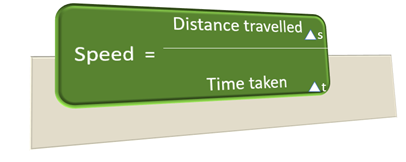### Velocity

Velocity is defined as the rate of change of an object’s position with respect to a frame of reference.

Velocity is a vector quantity as it describes both the magnitude and direction.

The SI derived unit for velocity is meter per second (m/s).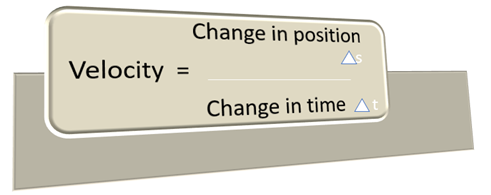### Acceleration

Acceleration is also a vector quantity and is defined as the rate of change of velocity with change in time.

The SI derived unit for acceleration is meter per second squared (m/s2).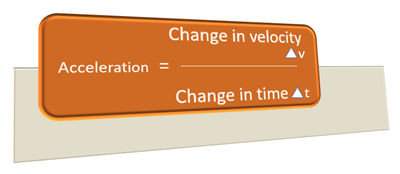### Time

A measurable period during which an action/event happens is Time.

The SI derived unit for Time is seconds (s).

### International System of Units (SI)

International system of units is the modern form of the Metric system. The units listed in this system are used as the standard units of measurements in almost every country in the world.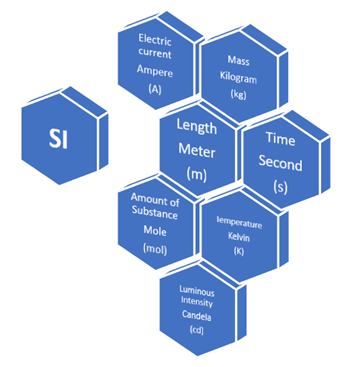## Unit conversions for Time

In this section we will look at different units used to measure Time and the conversion formulae needed to convert a value from one unit to another.

### Hour To Second (hr to s)### Minutes To Hour (min to hr)### Minutes To Day (min to days)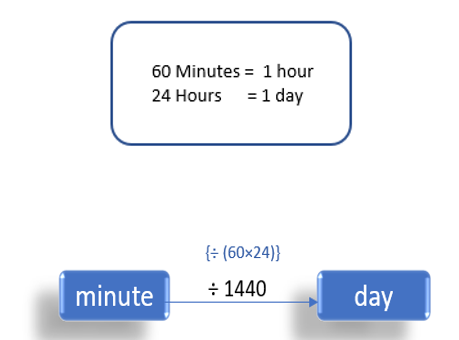### Days To Minute (days to min)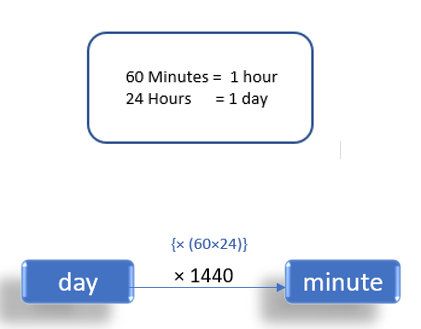### Hours To Days (hrs to days)### Seconds To Week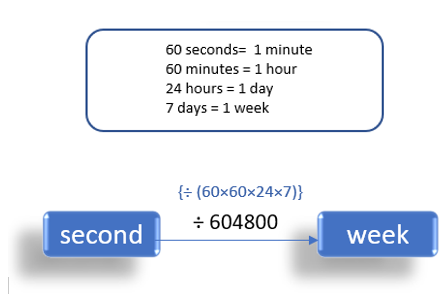## Unit conversions for Speed/Velocity

In this section, we will see various formulae for the conversion of values between different unit representations for speed/Velocity.

In general, the method used to arrive at the formula is based on the individual units in the numerator and the denominator.

### Meter/Second To kilometer/Hour (m/s to km/h)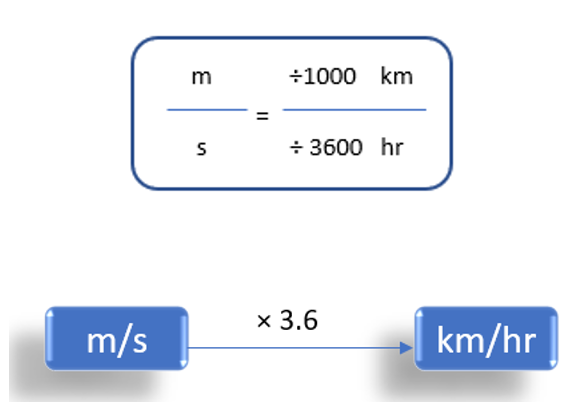### Kilometer/Hour To Meter/Second (km/h to m/s)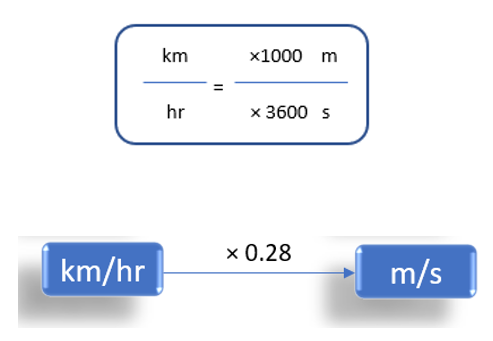### Kilometer/Second To Miles/Hour (km/s to mi/h)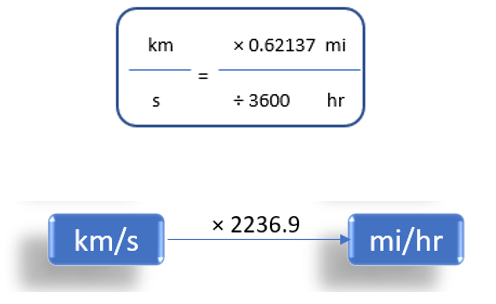### Feet/Second To Meter/Second (ft/s to m/s)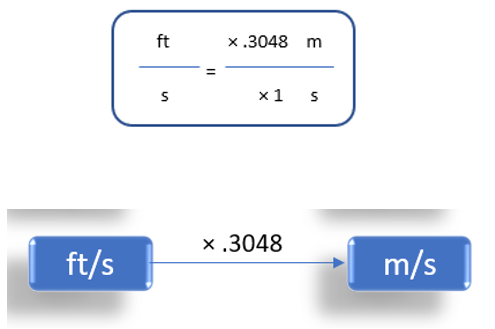### Miles/Hour To Meter/Second (mi/h to m/s)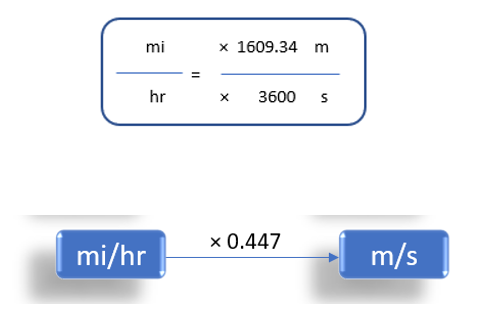### Meter/Second To Feet/Second (m/s to ft/s)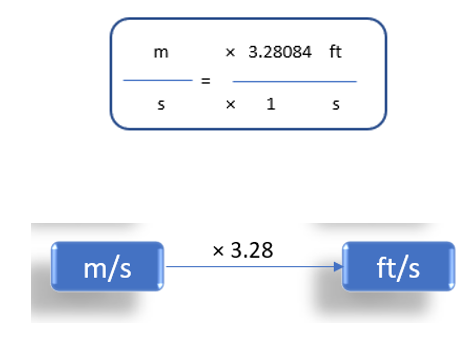### Kilometer/Second To Meter/Second (km/s to m/s)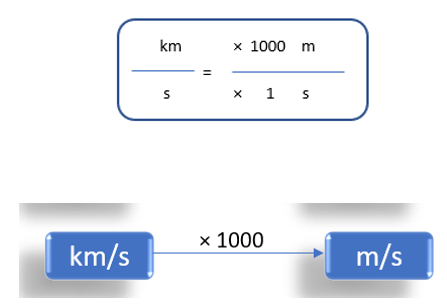### Centimeter/Second To Meter/Second (cm/s to m/s)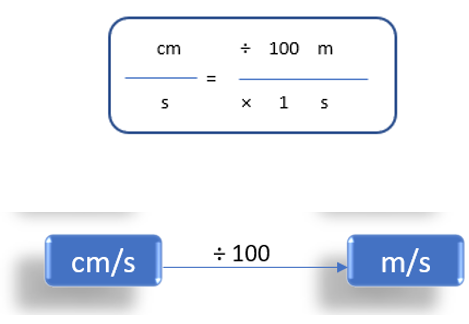### Feet/Minute To Meter/Second (ft/min to m/s)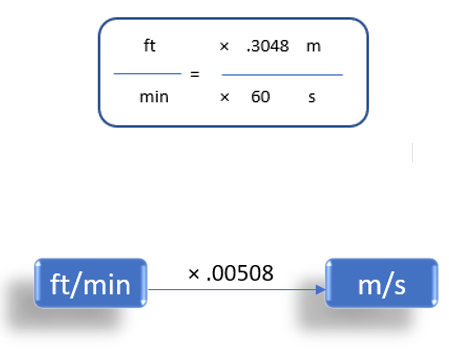### Miles/Hour To Feet/Second (mi/h to ft/s)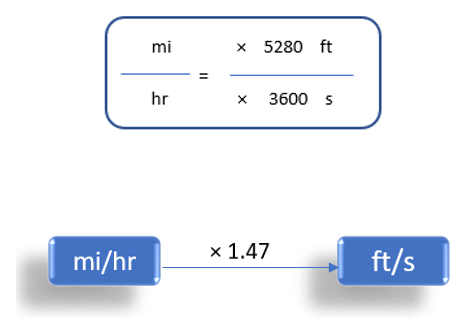### Rotations/Minute To Meter/Second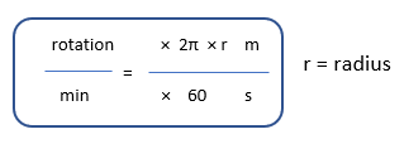where 2 × π × r = Linear Velocity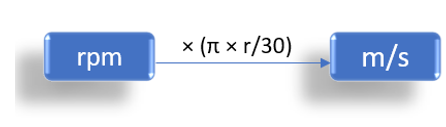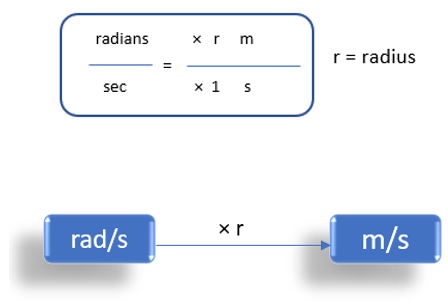### Meter/Second To Mach (m/s to Mach)

Mach is the ratio of the speed of a moving object through a fluid to the speed of sound in the same medium. Since it is a ratio, it does not have any dimension.

The speed of the sound is not constant. It varies depending on the temperature and the atmospheric pressure.## Unit conversions for Acceleration

In this section, we will see various formulae for the conversion between different unit representations for acceleration.

In general, the method used to arrive at the formula is based on the individual units in the numerator and the denominator.

### Feet/Second2 To Meter/Second2 (ft/s2 to m/s2)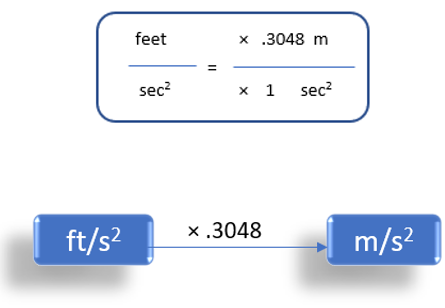### Meter/Second2 To Kilometer/Second2 (m/s2 to km/s2)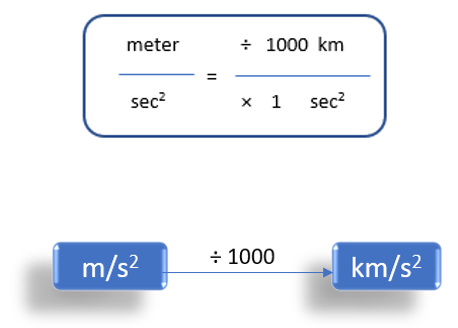### Meter/Second2 To Kilometer/Hour2 (m/s2 to km/h2)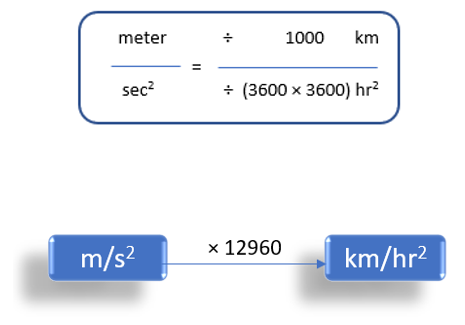### Feet/Second2 To Kilometer/Second2 (ft/s2 to km/s2)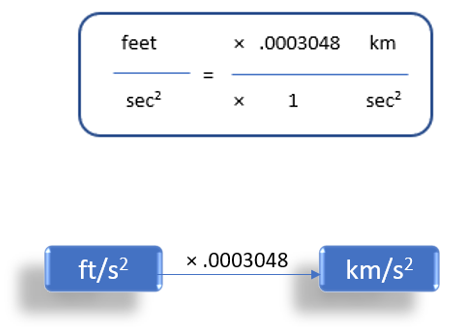### Kilometer/Second2 To Feet/Second2 (km/s2 to ft/s2)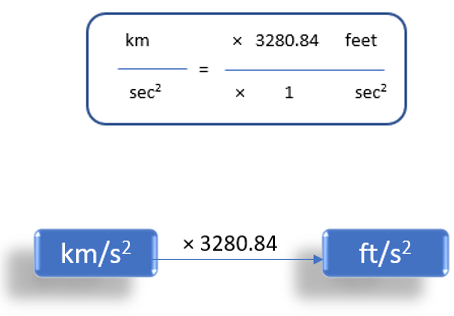### Kilometer/hour2 To Meter/Second2 (km/h2 to m/s2)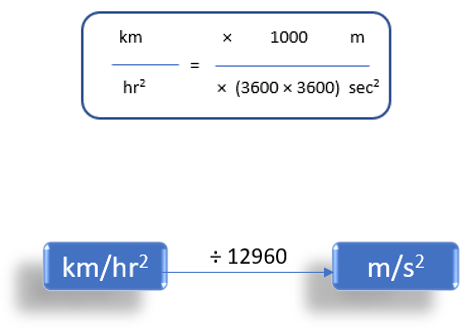### Meter/Second2 To Feet/Second2 (m/s2 to ft/s2)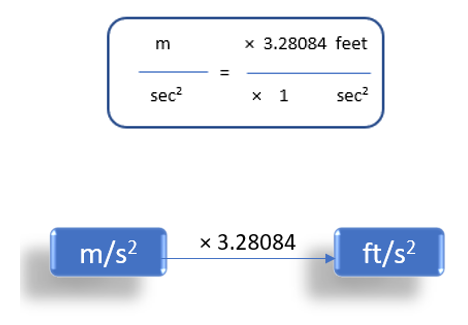### Miles/Second2 To Kilometer/Hour2 (mi/s2 to km/h2)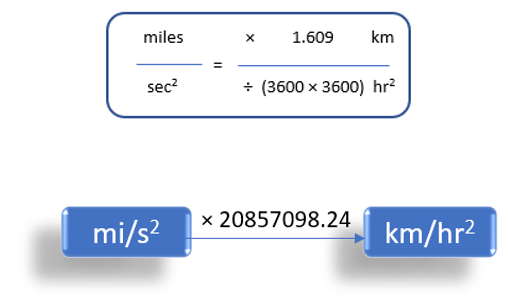### Kilometer/Hour2 To Miles/Hour2 (km/h2 to mi/h2)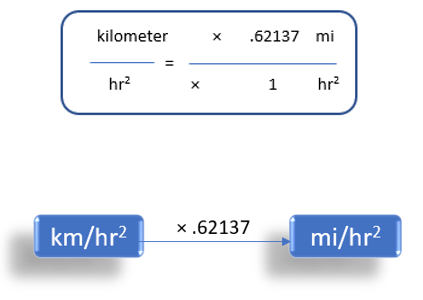### Gal To kilometer/hour2 (1 gal to km/h2)

1 Gal = 1 centimeter/second2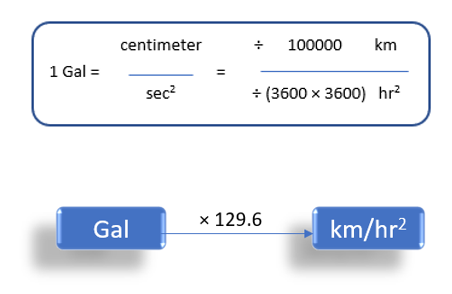## Examples

In this section we are going to see some examples for unit conversions.

 Example 1

Convert a speed of 90 meters per second to kilometer per hour

To convert a value from m/s to km/hr, we need to multiply it by 3.6

So, 90 m/s = 90 × 3.6 = 324 km/hr

 Example 2

Convert a speed of 10 feet per second to meters per second

To convert a value from ft/s to m/s, we need to multiply it by 0.3048

So, 10 ft/s = 10 × 0.3048 = 3.048 m/s

 Example 3

Convert a velocity of 25 mph to feet per second

To convert a value from miles/hr to feet/sec, we need to multiply it by 1.47

So, 25 mph = 25 × 1.47 = 36.7 ft/s

 Example 4

Convert an acceleration of 120 metre per second squared to kilometre per hour squared

To convert a value from meter/sec2 to kilometre/ hr2, we need to multiply it by 12960

So, 120 m/s2 = 120 × 12960 = 15,55,200 km/hr2

 Example 5

Convert 58 Gal into kilometer per hour squared

To convert a value from Gal to kilometre/ hr2, we need to multiply it by 129.6

So, 58 Gal = 58 × 129.6 = 7516.8 km/hr2

 Example 6

How many minutes are there in 4 days?

Hours in a day = 24

Hours in 4 days = 24 × 4 = 96

Minutes in 1 hour = 60

So, minutes in 96 hours = 96 × 60 = 5760

 Example 7

How many seconds are there in one week?

Number of days in 1 week = 7

Number of hours in a day = 24

Number of minutes in 1 hour = 60

Number of seconds in 1 minute = 60

So, number of seconds in 1 week = 60 × 60 × 24 × 7 = 604800

## Summary

In this article, we learnt about Time, Speed, Velocity and Acceleration. We learnt their definitions, units and the conversion rules/formulae between different units.

This may serve as a quick reference guide for any of the concepts mentioned above.

Written by Gayathri Sivasubramanian, Cuemath Teacher

## How many weeks are in 6 months?

Number of days in a year (12 months) = 365 (366 once every 4 years)

Number of days in a year (6 months) = 365/2 = 182.5

Number of days in a week = 7

So, the number of weeks in 6 months = 182.5/7 = 26.07 (approximately 26 weeks)

## What is the difference between Speed and Velocity?

Both speed and velocity are the ratio of the distance moved by an object to the time taken for the movement.

But Speed is a Scalar quantity. It considers only magnitude irrespective of the direction.

Whereas velocity is a Vector quantity and it represents both magnitude and direction.

## What is the relation between speed and acceleration?

Acceleration is the rate of change of speed. It denotes the change of speed with respect to time.

## How many seconds in an hour?

Number of seconds in a minute = 60

Number of minutes in an hour = 60

So, number of seconds in an hour = 60 × 60 = 3600

## How many minutes in a day?

Number of minutes in an hour = 60

Number of hours in a day = 24

So, number of minutes in a day = 60 × 24 = 1440